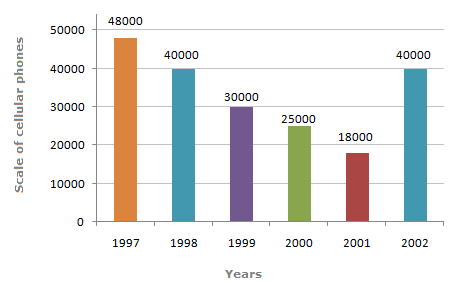# Data Interpretation - Bar Charts

Study the bar chart and answer the questions.

Sale of Cellular Phones1.

The difference in the sales of cellular phones for the years 1997 and 1999 is ?

 A. 500 units B. 1,000 units C. 5,000 units D. 18,000 units

Explanation:

The required answer is got by 48,000 - 30,000 = 18,000.

2.

The two years between which the rate of change of cellular phones is minimum are ?

 A. 1997 and 1998 B. 1999 and 2000 C. Both option (A) and (B) D. 2001 and 2002

Explanation:

The lowest rate of change for

For year 1997 and 1998 = ((48000 - 40000) / 40000) x 100 = 20%

For year 1999 and 2000 = ((30000 - 25000) / 25000) x 100 = 20%

is exhibited by both option (A) and (B).

3.

The sum of sales of cellular phones in the years 1999 and 2001 is equal to that in ?

 A. 1997 B. 1998 C. 2000 D. 2002

Explanation:

The sum of sales in the two years is 30,000 + 18,000 = 48,000, which is the sales value for 1997.

4.

The percentage increases in sales from 2001 to 2002 was ?

 A. 115 % B. 128 % C. 122 % D. 118 %